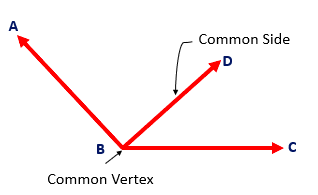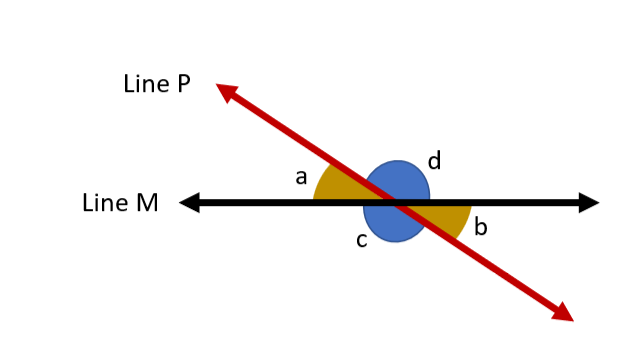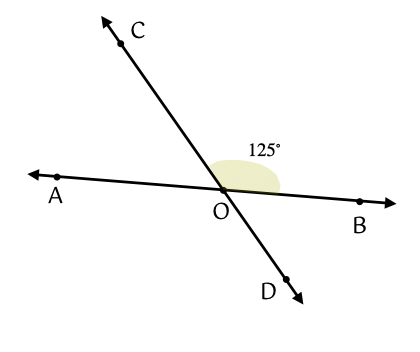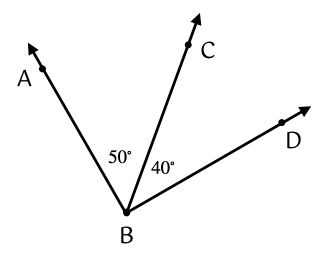• Adjacent Angles are two angles with a shared side and a common vertex.
• For example, in the diagram shown below, $\angle ABD$ and $\angle DBC$ are adjacent angles.• If the sum of a pair of adjacent angles is $90°$, then they are complementary to each other.
• If the sum of a pair of adjacent angles is $180°$, then they are supplementary to each other.

## Vertical Angles

• The pairs of opposite angles formed by intersecting lines are called Vertical Angles or Vertically Opposite Angles.
• For example, in the diagram shown below, $\angle a$ and $\angle b$ are vertical angles, and so are $\angle c$ and $\angle d$.• Vertical angles are always congruent. Hence, in the diagram above $m\angle a=m\angle b$, and $m\angle c=m\angle d$.

## Solved Examples

Question 1: Find the measure of $\angle AOD$, if $\angle BOC=125°$.Solution: $\angle AOD=\angle BOC$

$\angle AOD=125°$

Question 2: In the figure shown below, find the measure of $\angle ABD$.Solution: $\angle ABD=\angle ABC+\angle CBD$$=50°+40°$$=90°$

## Cheat Sheet

• Two angles having the same vertex and a common side between them are called Adjacent Angles.
• A pair of adjacent angles can be complementary or supplementary angles.
• Vertical angles are pairs of opposite angles formed by intersecting lines.
• Vertical angles are always equal in measure.

## Blunder Areas

• Vertical angles always are congruent.
• Vertical angles cannot be adjacent angles.
• Congruent angles have the same degree measure.